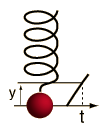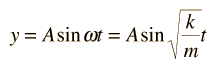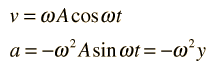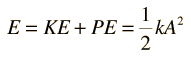Harmonic Motion

Simple harmonic motion is typified by the motion of a mass on a spring when it is subject to the linear elastic restoring force given by Hooke's Law. The motion is sinusoidal in time and demonstrates a single resonant frequency.Simple Harmonic Motion Equations

The motion equation for simple harmonic motion contains a complete description of the motion, and other parameters of the motion can be calculated from it.The velocity and acceleration are given by:The total energy for an undamped oscillator is the sum of its kinetic energy and potential energy which is constant at:Or, the way I remember it: